# A Appendix A - Lead Times

This appendix contains these topics:

## A.1 Introduction to Lead Times

Determining lead time is an essential part of any manufacturing or scheduling process. For any product that you purchase or manufacture, you encounter a time lag between when you order or start it and when you receive or finish it. To account for the lag, you must estimate the extra time and allow for it in your planning.

Cumulative lead time is the total amount of time that is required to produce a product. In process manufacturing, it is the time required to complete an entire process. The Shop Floor Control system uses the requested date of the order and, based on the level lead time for the product, calculates the appropriate order start date.

• Work order start dates

• Operation start and due dates

• Overlapping operations

• Overlapping and concurrent operations

• Manufacturing environment (assemble-to-order, make-to-order)

• Fixed or variable quantities

• Serial or overlap operations

• Fixed or variable time

• Number of shifts and operators

• Factoring by efficiency

• Protection

For any manufactured product, the system calculates four types of lead time:

• Level

• Manufacturing

• Cumulative

• Per unit

The Shop Floor Control system uses the following data in its calculation of lead times:

• Serial or overlap operations

• Fixed or variable lead time indicator

• Routing labor, setup, queue, move, and machine run hours

• Work center prime load code

• Number of employees or machines per work center

• Hours per work day

The system:

• Uses the information that you set up for each item on Plant Manufacturing Data in the Inventory Management system

• Coordinates the information with routing and work center information you enter in the Product Data Management (PDM) system

• Determines lead times for all parent and component items

At any point in your planning and scheduling process, you can change lead time values manually through Manufacturing Data.

You can use fixed or variable lead times for ingredients. The system subtracts fixed lead times directly from the requested date on the work request to calculate the start date of production. Fixed lead time remains the same regardless of the quantity produced. However, variable lead time adjusts according to the quantity produced.

The following explains important lead time concepts you need to understand.

Concept Description
Machine hours The number of machine hours required to produce the amount from the time basis code.
Labor hours The number of labor hours required to produce the amount from the time basis code.
Setup hours The number of hours required to prepare machinery to run a specific item, regardless of quantity.
Move hours The number of hours that a manufacturing work order is in transit from the completion of one operation to the beginning of the next.
Queue hours The number of hours that a job waits at a work center before setup or work is performed on the job. This value is the sum of the move hours and the queue hours.
Time basis code Indicates how machine or labor hours are expressed for a product. Time basis codes identify the time basis or rate to be used for machine or labor hours entered for every routing step, for example, 25 hours per 1,000 pieces or 15 hours per 10,000 pieces. You can maintain the time basis codes on Time Basis Codes. Time basis codes are user defined codes (30/TB).
Resource units Shows the available amount of capacity in a work center for the months in the calendar. For lead time purposes, as the system calculates the operation start and due dates, the system uses the available hours to calculate the operation start dates. You can maintain the resource units in Work Center Resource Units.
Prime load code Determines whether a work center is labor or machine intensive. The prime load code also determines whether the system uses the number of employees or number of machines to determine the daily resource units in the Resource Units table. You can maintain the prime load codes on Work Center Revisions. For calculating lead times, the following prime load code values are important:
• L = run labor hours

• M = machine labor hours

• B = run and setup hours

• C = machine and setup hours

Purchased parts You specify the level lead time, which is equal to the cumulative lead time. By default, the manufacturing lead time, lead time per unit, total queue/move hours, and setup times for purchased parts are zero.

## A.2 Work Order Start Dates

The system uses the level lead time or lead time per unit for an item defined on the Manufacturing Data screen to calculate the start date of a work order based on the order's due date.

When an item has a fixed lead time, the system uses its level lead time value in backscheduling to find the work order start date.

For example, the system generates a planned order for product 101 with a requested date of 10/15/17. The level lead time is 3 days for this product, so the system calculates the start date by counting back 3 working days on the shop floor calendar from (but not including) the requested date. The system assigns the order a start date of 10/12/17.

When an item has a variable lead time, the system uses the following calculation to determine the lead time days:

Figure A-1 Variable Lead Time Calculation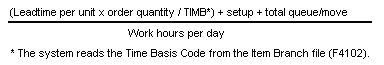Description of "Figure A-1 Variable Lead Time Calculation"

For example, to determine the start date, the system counts back the lead time days from the due date of planned orders. The system backschedules the due date, 10/15, 2 days to determine the start date of 10/13.

(32 x 1000/ 10,000) + 1 + 9) / 8

(3.2 + 10) / 8 = 2 days

The following table shows the values used in this example.

Field Value Used
Due date 10/15
Lead time per unit 32 hours
Order quantity 1000
Time Basis Code 10,000
Setup 1 hour
Total queue/move 9 hours
Work hours per day From manufacturing constants

## A.3 Operation Start and Due Dates

The system calculates the operation start and due dates with the average number of hours per operation.

If the work center resource calendar is not populated then all the operation start dates will be the same as the work order header start date.

If the work center resource calendar is populated then the hours are spread evenly across all the operations.

The system schedules the last operation due date on the day before the work order due date.

To determine operation start and due dates using variable lead times, the system schedules the actual hours from the work order routing instructions according to the same resource units rules for variable lead time.

The system uses the prime load code to determine what hours to use. The hours are then applied to the resource units table. The system applies queue time from the work order routing at the beginning of an operation, and applies move time at the end of an operation.

## A.4 Overlapping Operations

One method to compress lead times is to overlap operations. Overlapping operations are two or more operations in a routing that process at the same time. The percent of overlap is the amount of time that these operations can process concurrently. You can define at what point a second operation can begin before the first operation is complete. Because of setup, move, and queue times, the actual overlap in run time might be less than the percent of overlap defined.

In the following example, Operation B has a percent of overlap of 80%. This means that Operation B can begin when 80% of Operation A remains to be finished, or when Operation A is 20% complete. Operations A and B are both active as they overlap.

Figure A-2 Overlapping Processes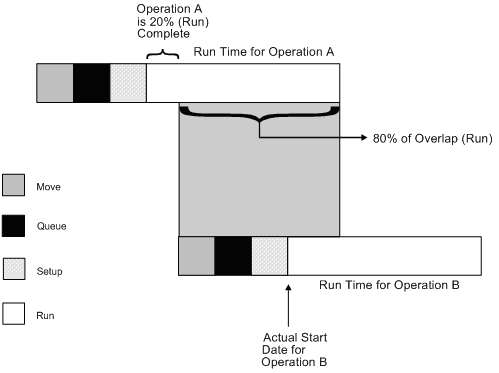Description of "Figure A-2 Overlapping Processes"

If the percent of overlap causes an operation to end later than the last operation in the routing, the system issues an error message and enters the work order start and requested dates into each operation.

## A.5 Overlapping and Concurrent Operations

If a percentage of overlap is specified in the Routing detail area, the work order routing includes specified operations that overlap. For example, an overlap percentage of 80% for an operation means that the next operation can start when 20% of the previous operation is complete.

Field Value
Work order complete date 05/01/17
Last operation 20 24 hours
First operation 10 24 hours
Resource hours per day-per work center 8 hours
Operation overlap on 20 75%

Operation Without overlap With overlap
Operation 10
start 04/27/17 04/27/17
complete 04/29/17 04/29/17
Operation 20
start 04/30/17 04/27/17
complete 05/02/17 04/30/17

Using the data from the above tables, the system advances the complete date of the previous operation by 75% of 24 or 18 hours. The system then recalculates the start date using the normal backscheduling rules. As a result, operations 10 and 20 overlap and will take 24 hours to complete. The following diagram illustrates this concept.

Date 4/27 4/28 4/29 4/30 5/1 5/2
Resource hours 8 8 8 8 8 8
OP 10 (24 hrs) <---- ----- ---->
OP 20 (24 hrs) (w/o overlap)       <---- ----- ---->
OP 10 (w/ overlap) <---- ----- ---->
OP 20 (w/ overlap) <- ----- ----- -->

The Leadtime Rollup program calculation updates the following within the Plant Manufacturing Data program:

• Total queue/move hours

• Setup hours

For a manufactured product, level lead time is the number of workdays required to complete the product once all items are available. Level lead time for a purchased item is the number of calendar days required for you to receive the item after the supplier receives your purchase order.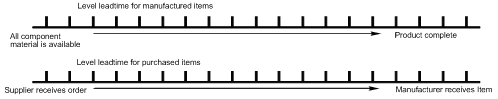Description of "Figure A-3 Level Lead Times"

#### A.6.1.1 Calculation

The system uses the following formula to calculate level lead time:

Figure A-4 Level Lead Time Formula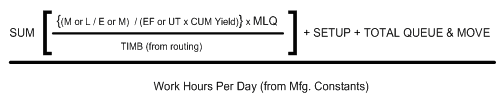Description of "Figure A-4 Level Lead Time Formula"

The following table defines the values used in the formula.

Value Description
SUM Sum of all operations
M or L Either machine or labor hours based on the prime load code
E or M Number of employees or machines in the work center
EF or UT Efficiency or Utilization from the work center
Cum Yield % From the Routing Master (F3003)
TIMB Time basis
Setup Setup time from the Routing Master (F3003)
Total Queue & Move Queue plus Move time from routing master or work center

For example:

Figure A-5 Level Lead Time Calculation with Values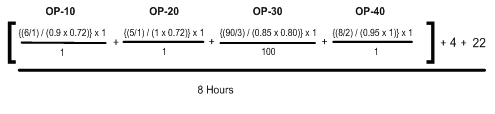Description of "Figure A-5 Level Lead Time Calculation with Values"

Figure A-6 Level Lead Time Calculated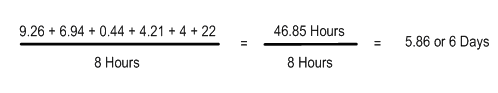Description of "Figure A-6 Level Lead Time Calculated"

Manufacturing lead time is the total number of workdays required to complete a product, from its lowest-level items to the final item, assuming all purchased items are in house, which includes:

• Order preparation time

• Queue time

• Setup time

• Run time

• Move time

• Inspection time

• Putaway time

And, it is the total of the level lead time for a product plus the longest manufacturing lead time of any of its items.

Lead times for purchased items are not included in manufacturing lead time calculations. The manufacturing lead time for a purchased item is its level lead time.

• Manufacturing leadtime for manufactured items

• Final Item

• Start product

#### A.6.2.1 Calculation

The system uses the following formula to calculate manufacturing lead time.

Item F Level LT = 1

Item G Level LT = 3

Item D Level LT = 4 Mfg LT = 4 + 0 = 4

Item E Level LT = 3 Mfg LT = 3 + 0 = 3

Item C Level LT = 4 Mfg LT = 4 + 4 = 8

Item B Level LT = 7 Mfg LT = 7 + 0 = 7

Item A Level LT = 2 Mfg LT = 2 + 8 = 10

Items A, B, C, D, and E are manufactured items. Items F and G are purchased items.

Cumulative lead time is the number of workdays that are required to acquire items and complete a product, from its lowest-level items to the final item, which is the level lead time for a product plus the longest cumulative lead time of any of its items. Unlike manufacturing lead time, cumulative lead time includes the lead times for purchased items. It covers both the time to acquire purchased items and the time to complete the product. The cumulative lead time for a purchased item is its level lead time.

Complete product

Start product

#### A.6.3.1 Calculation

The system uses the following formula to calculate cumulative lead time.

Item F Level LT = 1

Item G Level LT = 3

Item D Level LT = 4 Cume LT = 4 + 1 = 5

Item E Level LT = 3 Cume LT = 3 + 3 = 6

Item B Level LT = 7 Cume LT = 7 + 0 = 7

Item C Level LT = 4 Cume LT = 4 + 6 = 10

Item A Level LT = 2 Cume LT = 2 + 10 = 12

Items A, B, C, D, and E are manufactured items. Items F and G are purchased items.

### A.6.4 Per Unit Lead Time

Per unit lead time is the sum of the run times, as defined by the prime load codes for the work centers, factored by the routing time basis and converted to the lead time per unit. The per unit lead time sets valid start dates for orders planned in other than normal planned order quantity. When you run the leadtime rollup program, the system measures the per unit lead time in hours.

#### A.6.4.1 Calculation

The system uses the following formula to calculate per unit lead time.

Figure A-7 Per Unit Lead Time Formula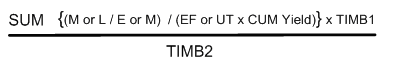Description of "Figure A-7 Per Unit Lead Time Formula"

The following table defines the values used in the formula.

Value Description
SUM Sum of all operations
M or L Machine or labor hours based on the prime load code
E or M Number of employees or machines from the work center
EF or UT Efficiency or Utilization from the work center
Cum Yield Cumulative Yield percentage from the routing
TIMB1 Time basis from Item Branch/Plant manufacturing data
TIMB2 Time basis from the Routing Master(F3003)

For example:

Figure A-8 Per Unit Lead Time Calculation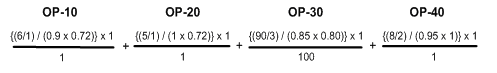Description of "Figure A-8 Per Unit Lead Time Calculation"

Figure A-9 Per Unit Lead Time Calculated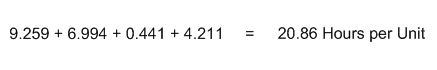Description of "Figure A-9 Per Unit Lead Time Calculated"

Although overlap is not shown in the formulae, it does have an effect on all of the leadtime calculations. For example, if OP-20 had 100% overlap, the 6.994 hours would be completed at the same time OP-10 is running, therefore reducing the leadtime per unit by 6.994 hours. Factoring in this overlap to all the preceding calculations, the results would be as follows:

• Level Lead Time = 4.99 or 5 days

• Manufacturing Lead Time = 6 days

• Cumulative Lead Time = 13 days

• Lead Time Per Unit = 13.91 hours